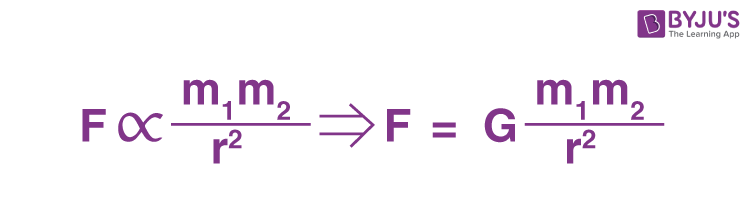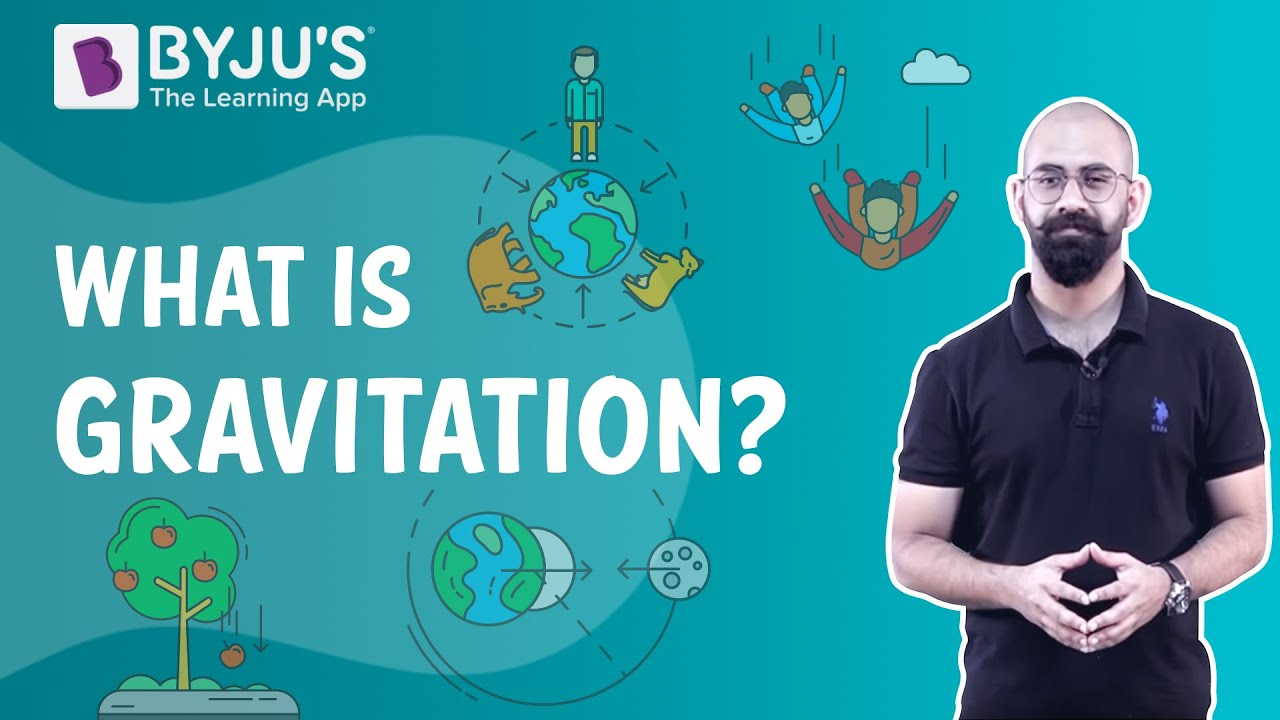# Difference Between Gravitation and Gravity

The terms Gravitation and Gravity define the phenomenon that causes masses to attract each other. These terms are used interchangeably, and it is permitted to do so. However, in some fields of study, the difference between gravitation and gravity is more prominent. The term ‘Gravity’ is used when the gravitational field is taken as a given, and the focus is on how a particular particle reacts to the field. In contrast, the term ‘Gravitation’ is used when the focus is the mutual attraction between pairs of masses.

## What is Gravitation?

Gravitation is the universal force of attraction that acts between all matter, big and small. It is the weakest of the fundamental forces in nature. However, Gravitation is well approximated by the Newton’s law of universal gravitation. Sir Issac Newton put forward the law of universal gravitation in 1687 and used it to explain the observed motion of the planets and moons.

Newton’s Law of Universal Gravitation states that every particle attracts every other particle in the universe with force directly proportional to the product of the masses and inversely proportional to the square of the distance between them.

The universal gravitation equation thus takes the form:F is the gravitational force between bodies, m1 and m2 are masses of bodies, r is the distance between the centres of the bodies, G is the universal gravitational constant. The value of G is 6.67×10-11 Nm2/ Kg2.

The Universal Gravitational Law can explain almost anything, right from how an apple falls from a tree to why the moon revolves around the earth. Watch the video and understand the beauty of the law of universal gravitation.## What is Gravity?

Essentially, the term ‘gravity’ has a similar meaning as ‘gravitation.’ However, the term ‘gravity’ is generally attached to an object, for example, ‘gravity of the earth,’ ‘gravity of the moon, Mars, Sun,’ etc. It represents the gravitational force exerted by an object. So, to summarize, we can say that gravitation and gravity have a cause-effect relationship; gravitation, or the gravitational force, is the cause, and gravity is its effect.

### Earth’s Gravity

On Earth, all bodies experience a downward force of gravity which Earth’s mass exerts on them. The Earth’s gravity is measured by the acceleration of the freely falling objects. At Earth’s surface, the acceleration of gravity is 9.8 ms-2. Thus, for every second an object is in free fall, its speed increases by about 9.8 metres per second. The acceleration due to Earth’s gravity is denoted by the symbol ‘g.’

Read More: Acceleration Due to Gravity

Watch the video and understand how the acceleration due to the gravity of Earth varies with altitude.Test your knowledge on Gravitation and gravity differences

1. Vaibhavi Bhardwaj

This is very nice app I just love it.
And it also improve my mark in exam.

2. Kshitiz karki

Hello
It helped me a lot

3. Siddharth Paul

Very good app helped me a lot for exams

4. Bhoomika Venkatesh

I love byjus

5. Dibya Uranw

It is very helpful for us, thanks for help

6. Nandini

Thank you so much! Very helpful.

7. i really like it